# Plotting With Perl 6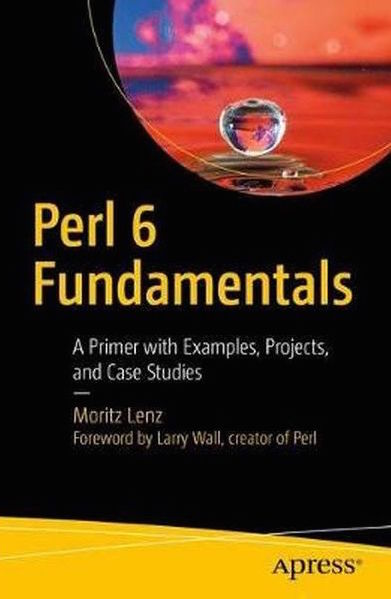(This chapter originally appeared in Perl 6 Fundamentals: A Primer with Examples, Projects, and Case Studies, by Moritz Lenz, published 2017 by Apress Media, LLC. Reprinted with permission.)

Occasionally I come across git repositories where I want to know how active they are and who the main developers are.

Let’s develop a script that plots the commit history, and explore how to use Python modules in Perl 6.

## Extracting the Stats

We want to plot the number of commits by author and date. We can get this information easily by passing some options to `git log`:

``````my \$proc = run :out, <git log --date=short --pretty=format:%ad!%an>;
my (%total, %by-author, %dates);
for \$proc.out.lines -> \$line {
my ( \$date, \$author ) = \$line.split: '!', 2;
%total{\$author}++;
%by-author{\$author}{\$date}++;
%dates{\$date}++;
}``````

`run` executes an external command and `:out` tells it to capture the command’s output, making it available as `\$proc.out`. The command is a list, with the first element being the actual executable and the rest of the elements are command line arguments to this executable.

Here `git log` gets the options `--date short --pretty=format:%ad!%an`, which instructs it to produce lines like `2017-03-01!John Doe`. This line can be parsed with a simple call to `\$line.split: '!', 2`, which splits on the `!` and limits the result to two elements. Assigning it to a two-element list `( \$date, \$author )` unpacks it. We then use hashes to count commits by author (in `%total`), by author and date (`%by-author`), and finally by date. In the second case, `%by-author{\$author}` isn’t even a hash yet and we can still hash-index it. This is due to a feature called autovivification, which automatically creates (“vivifies”) objects where we need them. The use of `++` creates integers, `{...}` indexing creates hashes, `[...]` indexing, `.push` creates arrays, and so on.

To get from these hashes to the top contributors by commit count, we can sort `%total` by value. Since this sorts in ascending order, sorting by the negative value returns the list in descending order. The list contains Pair objects, where we only want the first five, and only their keys:

``my @top-authors = %total.sort(-*.value).head(5).map(*.key);``

For each author, we can extract the dates of their activity and their commit counts like this:

``````my @dates  = %by-author{\$author}.keys.sort;
my @counts = %by-author{\$author}{@dates};``````

The last line uses slicing, that is, indexing a hash with a list to return a list of elements.

## Plotting with Python

Matplotlib is a very versatile library for all sorts of plotting and visualization tasks. It is based on NumPy, a Python library for scientific and numeric computing.

Matplotlib is written in Python and for Python programs, but that won’t stop us from using it in a Perl 6 program.

But first, let’s take a look at a basic plotting example that uses dates on the x-axis:

``````import datetime
import matplotlib.pyplot as plt

fig, subplots = plt.subplots()
subplots.plot(
[datetime.date(2017, 1, 5), datetime.date(2017, 3, 5), datetime.date(2017, 5, 5)],
[ 42, 23, 42 ],
label='An example',
)
fig.autofmt_xdate()
plt.show()``````

To make this run, you have to install Python 2.7 and matplotlib1. You can do this on Debian-based Linux systems with `apt-get install -y python-matplotlib`. The package name is the same on RPM-based distributions such as CentOS or SUSE Linux. MacOS users are advised to install Python 2.7 through homebrew and macports and then use `pip2 install matplotlib` or ```pip2.7 install matplotlib``` to get the library. Windows installation is probably easiest through the conda package manager, which offers pre-built binaries of both Python and matplotlib.

When you run this script with `python2.7 dates.py`, it opens a GUI window, showing the plot and some controls, which allow you to zoom, scroll, and write the plot graphic to a file: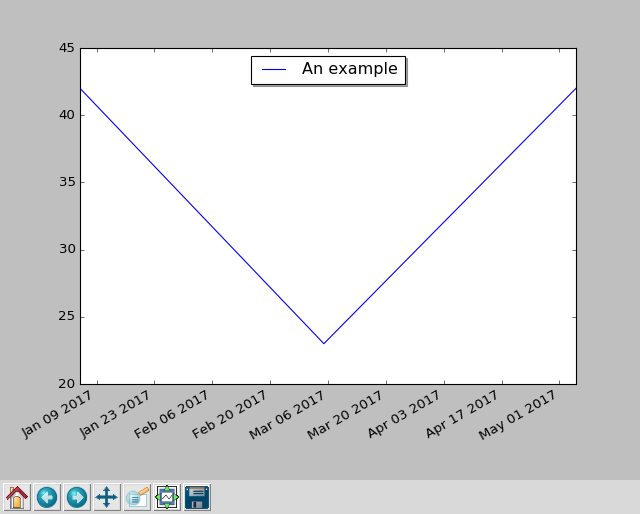## Bridging the Gap

The Rakudo Perl 6 compiler comes with a handy library for calling foreign functions – called `NativeCall` – which allows you to call functions written in C, or anything with a compatible binary interface.

The Inline::Python library uses the native call functionality to talk to Python’s C API and offers interoperability between Perl 6 and Python code. At the time of writing, this interoperability is still fragile in places, but can be worth using for some of the great libraries that Python has to offer.

To install `Inline::Python`, you must have a C compiler available, and then run

``````\$ zef install Inline::Python
``````

Now you can start to run Python 2 code in your Perl 6 programs:

``````use Inline::Python;

my \$py = Inline::Python.new;
\$py.run: 'print("Hello, Perl 6")';``````

Besides the `run` method, which takes a string of Python code and executes it, you can also use `call` to call Python routines by specifying the namespace, the routine to call, and a list of arguments:

``````use Inline::Python;

my \$py = Inline::Python.new;
\$py.run('import datetime');
my \$date = \$py.call('datetime', 'date', 2017, 1, 31);
\$py.call('__builtin__', 'print', \$date);    # 2017-01-31``````

The arguments that you pass to `call` are Perl 6 objects, such as the three `Int` objects in this example. `Inline::Python` automatically translates them into the corresponding Python built-in data structure. It translates numbers, strings, arrays, and hashes. Return values are also translated in the opposite direction, though since Python 2 does not distinguish properly between byte and Unicode strings, Python strings end up as buffers in Perl 6.

Objects that `Inline::Python` cannot translate are handled as opaque objects on the Perl 6 side. You can pass them back into Python routines (as shown with the `print` call above) and you can call methods on them:

``say \$date.isoformat().decode;               # 2017-01-31``

Perl 6 exposes attributes through methods, so Perl 6 has no syntax for accessing attributes from foreign objects directly. For instance, if you try to access the `year` attribute of `datetime.date` through the normal method call syntax, you get an error:

``say \$date.year;``

dies with

``````'int' object is not callable
``````

Instead, you have to use the `getattr` builtin:

``say \$py.call('__builtin__', 'getattr', \$date, 'year');``

## Using the Bridge to Plot

We need access to two namespaces in Python, `datetime` and `matplotlib.pyplot`, so let’s start by importing them and writing some short helpers:

``````my \$py = Inline::Python.new;
\$py.run('import datetime');
\$py.run('import matplotlib.pyplot');
sub plot(Str \$name, |c) {
\$py.call('matplotlib.pyplot', \$name, |c);
}

sub pydate(Str \$d) {
\$py.call('datetime', 'date', \$d.split('-').map(*.Int));
}``````

We can now call `pydate('2017-03-01')` to create a Python `datetime.date` object from an ISO-formatted string and call the `plot` function to access functionality from matplotlib:

``````my (\$figure, \$subplots) = plot('subplots');
\$figure.autofmt_xdate();

my @dates = %dates.keys.sort;
\$subplots.plot:
\$[@dates.map(&pydate)],
\$[ %dates{@dates} ],
label     => 'Total',
marker    => '.',
linestyle => '';``````

The Perl 6 call `plot('subplots')` corresponds to the Python code `fig, subplots = plt.subplots()`. Passing arrays to Python functions needs a bit of extra work, because `Inline::Python` flattens arrays. Using an extra `\$` sigil in front of an array puts it into an extra scalar and thus prevents the flattening.

Now we can actually plot the number of commits by author, add a legend, and plot the result:

``````for @top-authors -> \$author {
my @dates = %by-author{\$author}.keys.sort;
my @counts = %by-author{\$author}{@dates};
\$subplots.plot:
\$[ @dates.map(&pydate) ],
\$@counts,
label     => \$author,
marker    =>'.',
linestyle => '';
}

plot('title', 'Contributions per day');
plot('show');``````

When run in the zef git repository, it produces this plot: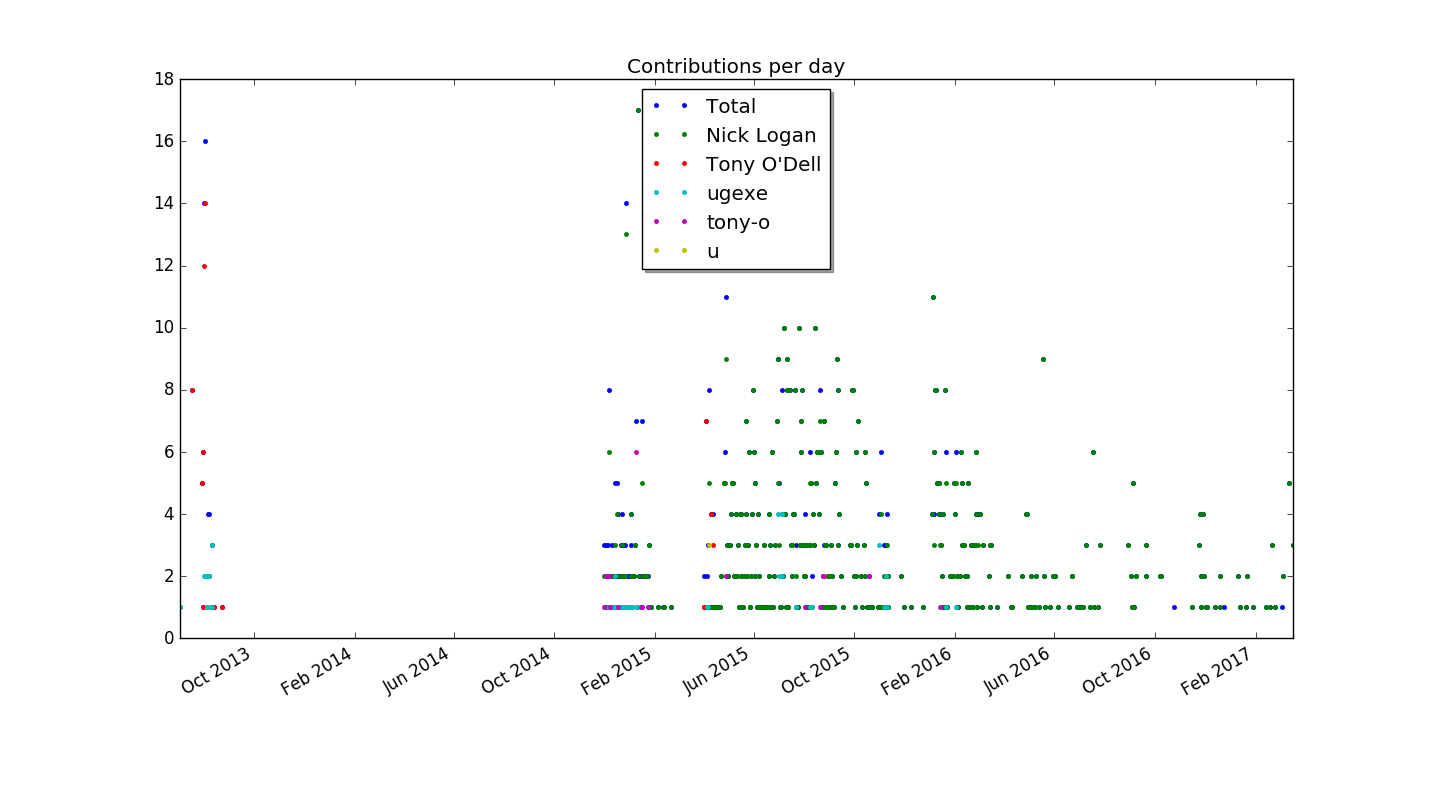## Stacked Plots

I am not yet happy with the plot, so I want to explore using stacked plots for presenting the same information. In a regular plot, the y-coordinate of each plotted value is proportional to its value. In a stacked plot, it is the distance to the previous value that is proportional to its value. This is nice for values that add up to a total that is also interesting.

Matplotlib offers a method called `stackplot` for this task. Contrary to multiple `plot` calls on a subplot object, it requires a shared x-axis for all data series. Hence we must construct one array for each author of git commits, where dates with no value are set to zero.

This time we have to construct an array of arrays where each inner array has the values for one author:

``````my @dates = %dates.keys.sort;
my @stack = \$[] xx @top-authors;

for @dates -> \$d {
for @top-authors.kv -> \$idx, \$author {
@stack[\$idx].push: %by-author{\$author}{\$d} // 0;
}
}``````

Now plotting becomes a simple matter of a method call, followed by the usual commands to add a title and show the plot:

``````\$subplots.stackplot(\$[@dates.map(&pydate)], @stack);
plot('title', 'Contributions per day');
plot('show');``````

The result (again run on the zef source repository) is this: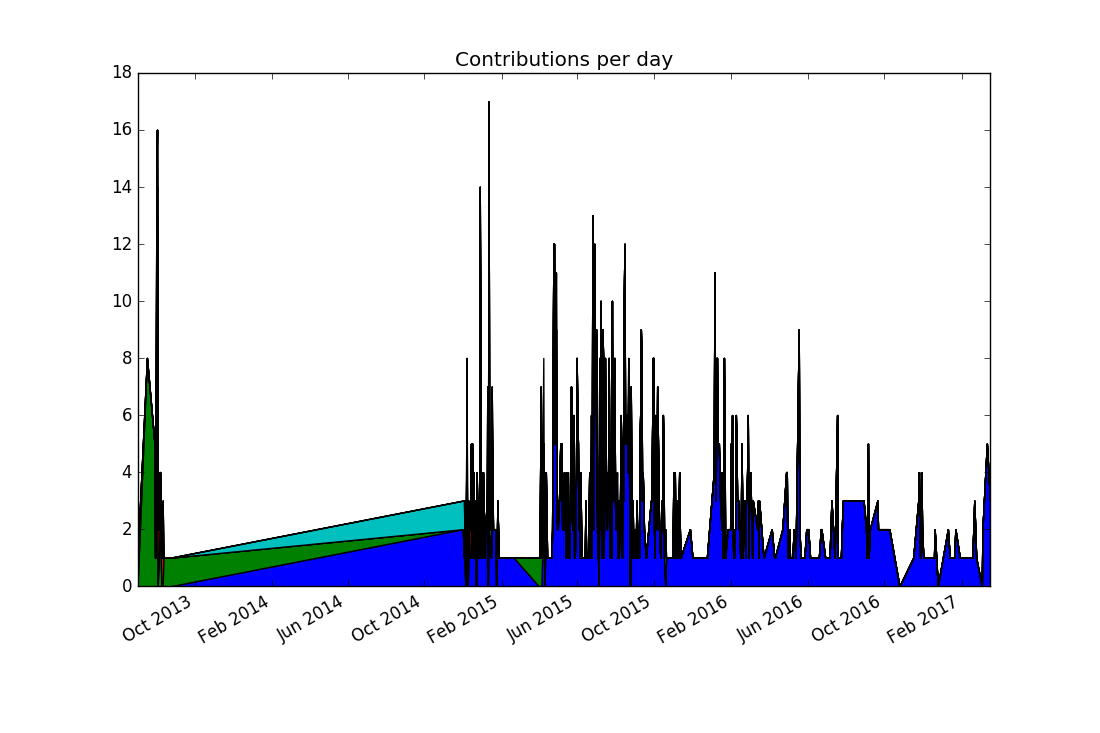Comparing this to the previous visualization reveals a discrepancy: There were no commits in 2014, and yet the stacked plot makes it appear this way. In fact, the previous plots would have shown the same “alternative facts” if we had chosen lines instead of points. It comes from matplotlib (like nearly all plotting libraries) interpolates linearly between data points. But in our case, a date with no data points means zero commits happened on that date.

To communicate this to matplotlib, we must explicitly insert zero values for missing dates. This can be achieved by replacing

``my @dates = %dates.keys.sort;``

with the line

``my @dates = %dates.keys.minmax;``

The `minmax` method finds the minimal and maximal values, and returns them in a Range. Assigning the range to an array turns it into an array of all values between the minimal and the maximal value. The logic for assembling the `@stack` variable already maps missing values to zero.

The result looks a bit better, but still far from perfect: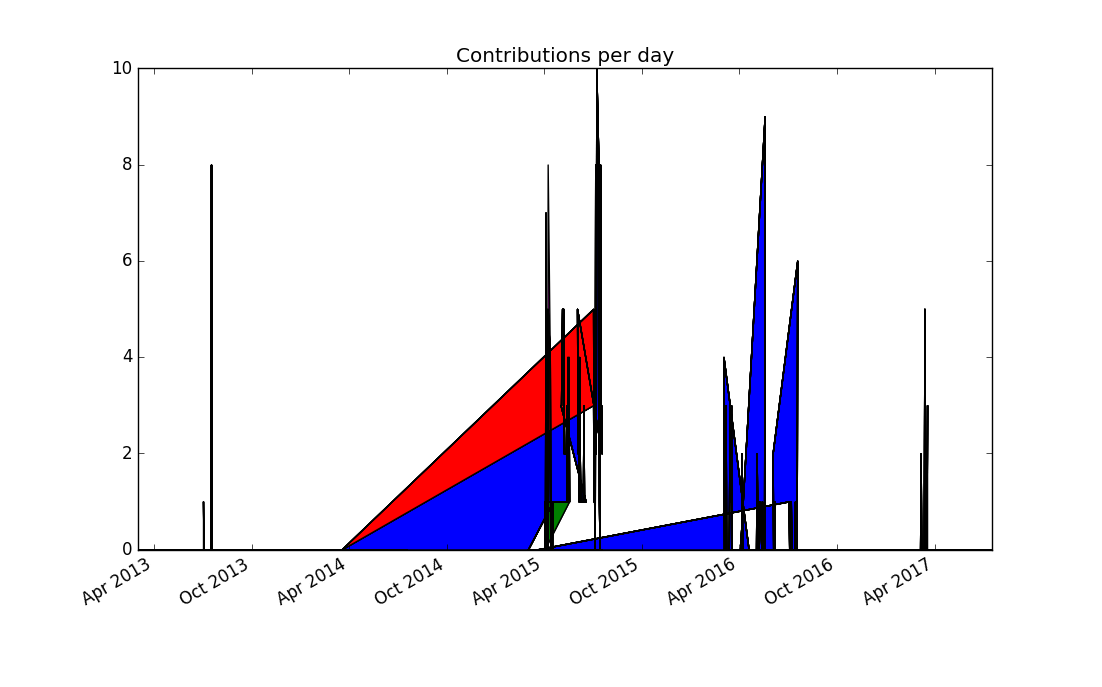Thinking more about the problem, contributions from separate days should not be joined together, because it produces misleading results. Matplotlib doesn’t support adding a legend automatically to stacked plots, so this seems to be to be a dead end.

Since a dot plot didn’t work very well, let’s try a different kind of plot that represents each data point separately: a bar chart, or more specifically, a stacked bar chart. Matplotlib offers the `bar` plotting method where the named parameter `bottom` can be used to generate the stacking:

``````my @dates = %dates.keys.sort;
my @stack = \$[] xx @top-authors;
my @bottom = \$[] xx @top-authors;

for @dates -> \$d {
my \$bottom = 0;
for @top-authors.kv -> \$idx, \$author {
@bottom[\$idx].push: \$bottom;
my \$value = %by-author{\$author}{\$d} // 0;
@stack[\$idx].push: \$value;
\$bottom += \$value;
}
}``````

We need to supply color names ourselves and set the edge color of the bars to the same color, otherwise the black edge color dominates the result:

``````my \$width = 1.0;
my @colors = <red green blue yellow black>;
my @plots;

for @top-authors.kv -> \$idx, \$author {
@plots.push: plot(
'bar',
\$[@dates.map(&pydate)],
@stack[\$idx],
\$width,
bottom => @bottom[\$idx],
color => @colors[\$idx],
edgecolor => @colors[\$idx],
);
}
plot('legend', \$@plots, \$@top-authors);

plot('title', 'Contributions per day');
plot('show');``````

This produces the first plot that’s actually informative and not misleading (provided you’re not color blind):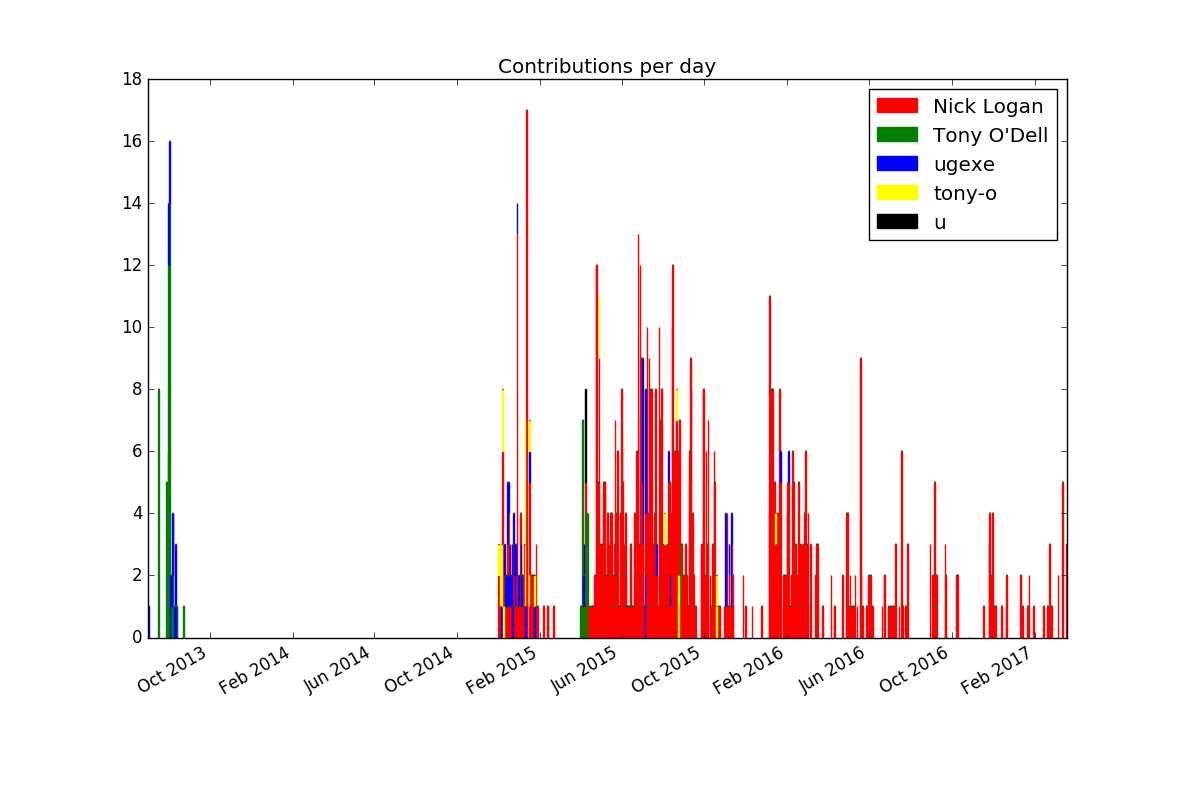If you want to improve the result further, you could experiment with limiting the number of bars by lumping together contributions by week or month (or maybe `\$n`-day period).

## Idiomatic Use of Inline::Python

Now that the plots look informative and correct, it’s time to explore how to better emulate the typical Python APIs through `Inline::Python`.

### Types of Python APIs

Python is an object-oriented language, so many APIs involve method calls, which `Inline::Python` helpfully automatically translates for us.

But the objects must come from somewhere and typically this is by calling a function that returns an object, or by instantiating a class. In Python, those two are really the same under the hood, since instantiating a class is the same as calling the class as if it were a function.

An example of this (in Python) would be

``````from matplotlib.pyplot import subplots
result = subplots()``````

But the matplotlib documentation tends to use another, equivalent syntax:

``````import matplotlib.pyplot as plt
result = plt.subplots()``````

This uses the `subplots` symbol (class or function) as a method on the module `matplotlib.pyplot`, which the import statement aliases to `plt`. This is a more object-oriented syntax for the same API.

### Mapping the Function API

The previous code examples used this Perl 6 code to call the `subplots` symbol:

``````my \$py = Inline::Python.new;
\$py.run('import matplotlib.pyplot');
sub plot(Str \$name, |c) {
\$py.call('matplotlib.pyplot', \$name, |c);
}

my (\$figure, \$subplots) = plot('subplots');``````

If we want to call `subplots()` instead of `plot('subplots')`, and `bar(args)` instead of `plot('bar', args)`, we can use a function to generate wrapper functions:

``````my \$py = Inline::Python.new;

sub gen(Str \$namespace, *@names) {
\$py.run("import \$namespace");

return @names.map: -> \$name {
sub (|args) {
\$py.call(\$namespace, \$name, |args);
}
}
}

my (&subplots, &bar, &legend, &title, &show)
= gen('matplotlib.pyplot', <subplots bar legend title show>);

my (\$figure, \$subplots) = subplots();

# more code here

legend(\$@plots, \$@top-authors);
title('Contributions per day');
show();``````

This makes the functions’ usage quite nice, but comes at the cost of duplicating their names. One can view this as a feature, because it allows the creation of different aliases, or as a source for bugs when the order is messed up, or a name misspelled.

How could we avoid the duplication should we choose to create wrapper functions?

This is where Perl 6’s flexibility and introspection abilities pay off. There are two key components that allow a nicer solution: the fact that declarations are expressions, and that you can introspect variables for their names.

The first part means you can write `mysub my (\$a, \$b)`, which declares the variables `\$a` and `\$b`, and calls a function with those variables as arguments. The second part means that `\$a.VAR.name` returns a string `'\$a'`, the name of the variable.

Let’s combine this to create a wrapper that initializes subroutines for us:

``````sub pysub(Str \$namespace, |args) {
\$py.run("import \$namespace");

for args <-> \$sub {
my \$name = \$sub.VAR.name.substr(1);
\$sub = sub (|args) {
\$py.call(\$namespace, \$name, |args);
}
}
}

pysub 'matplotlib.pyplot',
my (&subplots, &bar, &legend, &title, &show);``````

This avoids duplicating the name, but forces us to use some lower-level Perl 6 features in sub `pysub`. Using ordinary variables means that accessing their `.VAR.name` results in the name of the variable, not the name of the variable that’s used on the caller side. So we can’t use slurpy arguments as in

``sub pysub(Str \$namespace, *@subs)``

Instead we must use `|args` to obtain the rest of the arguments in a Capture. This doesn’t flatten the list of variables passed to the function, so when we iterate over them, we must do so by accessing `args`. By default, loop variables are read-only, which we can avoid by using `<->` instead of `->` to introduce the signature. Fortunately, that also preserves the name of the caller side variable.

### An Object-Oriented Interface

Instead of exposing the functions, we can also create types that emulate the method calls on Python modules. For that we can implement a class with a method `FALLBACK`, which Perl 6 calls for us when calling a method that is not implemented in the class:

``````class PyPlot is Mu {
has \$.py;
submethod TWEAK {
\$!py.run('import matplotlib.pyplot');
}
method FALLBACK(\$name, |args) {
\$!py.call('matplotlib.pyplot', \$name, |args);
}
}

my \$pyplot = PyPlot.new(:\$py);
my (\$figure, \$subplots) = \$pyplot.subplots;
# plotting code goes here
\$pyplot.legend(\$@plots, \$@top-authors);

\$pyplot.title('Contributions per day');
\$pyplot.show;``````

Class `PyPlot` inherits directly from `Mu`, the root of the Perl 6 type hierarchy, instead of `Any`, the default parent class (which in turn inherits from `Mu`). `Any` introduces a large number of methods that Perl 6 objects get by default and since `FALLBACK` is only invoked when a method is not present, this is something to avoid.

The method `TWEAK` is another method that Perl 6 calls automatically for us, after the object has been fully instantiated. All-caps method names are reserved for such special purposes. It is marked as a `submethod`, which means it is not inherited into subclasses. Since `TWEAK` is called at the level of each class, if it were a regular method, a subclass would call it twice implicitly. Note that `TWEAK` is only supported in Rakudo version 2016.11 and later.

There’s nothing specific to the Python package `matplotlib.pyplot` in class `PyPlot`, except the namespace name. We could easily generalize it to any namespace:

``````class PythonModule is Mu {
has \$.py;
has \$.namespace;
submethod TWEAK {
\$!py.run("import \$!namespace");
}
method FALLBACK(\$name, |args) {
\$!py.call(\$!namespace, \$name, |args);
}
}

my \$pyplot = PythonModule.new(:\$py, :namespace<matplotlib.pyplot>);``````

This is one Perl 6 type that can represent any Python module. If instead we want a separate Perl 6 type for each Python module, we could use roles, which are optionally parameterized:

``````role PythonModule[Str \$namespace] is Mu {
has \$.py;
submethod TWEAK {
\$!py.run("import \$namespace");
}
method FALLBACK(\$name, |args) {
\$!py.call(\$namespace, \$name, |args);
}
}

my \$pyplot = PythonModule['matplotlib.pyplot'].new(:\$py);``````

Using this approach, we can create type constraints for Python modules in Perl 6 space:

``````sub plot-histogram(PythonModule['matplotlib.pyplot'], @data) {
# implementation here
}``````

Passing in any wrapped Python module other than `matplotlib.pyplot` results in a type error.

## Summary

We’ve explored several ways to represent commit occurrence in plots and utilized `Inline::Python` to interface with a Python based plotting library.

A bit of Perl 6 meta programming allowed us to emulate different kinds of Python APIs pretty directly in Perl 6 code, allowing a fairly direct translation of the original library’s documentation into Perl 6 code.

1: The reason why Python 2.7 has to be used is that, at the time of writing, `Inline::Python` does not yet support Python 3.